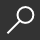## Propagation of Waves and the Equations of Hydrodynamics by Jacques Hadamard and D. Delphenich

Overview -

This seminal work of Hadamard on the mathematical theory of waves was written over 100 years ago and it continues to be cited as a reference by researchers in mathematical physics.

The reason for the enduring interest in this book can be found in its legacy. The conception of waves as discontinuities in some level of derivative of a wave function that propagate along the bicharacteristics of the wave equation spawned many of the important advances to both the purely mathematical theory of hyperbolic equations, as well as the more physical and engineering-oriented treatments of the subject of wave motion.

In mathematics, one can follow the implications of this work into the subsequent lectures that Hadamard gave on the Cauchy problem for linear partial differential equations. One should, however, regard this masterful treatise not only as a precursor to the later lectures on the Cauchy problem, but as a complementary work in which he establishes the roots of the mathematical theory in continuum mechanics.

local_shippingFor DeliveryOn Order. Usually ships in 2-4 weeks
This item is Non-Returnable.
FREE Shipping for Club Members help## More About Propagation of Waves and the Equations of Hydrodynamics by Jacques Hadamard; D. Delphenich

### Overview

This seminal work of Hadamard on the mathematical theory of waves was written over 100 years ago and it continues to be cited as a reference by researchers in mathematical physics.

The reason for the enduring interest in this book can be found in its legacy. The conception of waves as discontinuities in some level of derivative of a wave function that propagate along the bicharacteristics of the wave equation spawned many of the important advances to both the purely mathematical theory of hyperbolic equations, as well as the more physical and engineering-oriented treatments of the subject of wave motion.

In mathematics, one can follow the implications of this work into the subsequent lectures that Hadamard gave on the Cauchy problem for linear partial differential equations. One should, however, regard this masterful treatise not only as a precursor to the later lectures on the Cauchy problem, but as a complementary work in which he establishes the roots of the mathematical theory in continuum mechanics.

This item is Non-Returnable.

### Details

• ISBN-13: 9783764399689
• ISBN-10: 3764399686
• Publisher: Birkhauser Basel
• Publish Date: January 2011
• Page Count: 300

Series: Progress in Mathematical Physics #57Related Categories